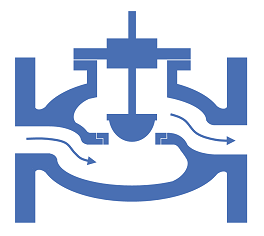# Summary

Cv and Kv are singles values in units of flowrate that may be used to characterise the relationship between flowrate and pressure loss for fittings and equipment. This article demonstrates how to calculate the Cv or Kv values, and how to use these values to determine the pressure loss for a given flowrate.

# Definitions

 : Flow coefficient determined on a US customary basis : Flow coefficient determined on a metric basis : Flowrate of fluid : Specific gravity of the fluid : Pressure change through the equipment : Density of the fluid

# Introduction

There are many ways of describing the pressure loss through pipes, fittings and equipment. The Cv and Kv methods use a single number to characterise the flowrate through the equipment at a known pressure loss, this number may then be used to estimate the pressure loss characteristics of the equipment at other flowrates.While the Cv and Kv methods may be used to describe many types of fitting and equipment, the most common use is for describing the flow characteristics of control valves. The Cv value is in units of US gallons per minute, while the Kv value is in units of m3/hr. The Cv values, using US customary units are far more widely reported than Kv values, using Metric units.

# Calculation of Pressure Loss using the Cv Method

A Cv value is defined as the rate of flow of water in US gallons per Minute at 60°F at a pressure drop of 1 psi across the equipment. Cv relates to pressure drop and flowrate via the following expression (imperial units):

Where Q is in USGPM and ΔP is in psi.

Thus for a given Cv, SG and Q the pressure drop across an item of equipment is calculated as:

Cv relates to pressure drop and flow rate via the following expression (Metric Units):

Where Q is in L/min, ρ is in kg/m3 and ΔP is in bar.

Thus for a given Cv, ρ and Q the pressure drop across an item of equipment is calculated as:

# Calculation of Pressure Loss using the Kv Method

A Kv value is defined as the rate of flow of water in m3/hr and 4°C at a pressure drop of 1 bar across the equipment. Kv relates to pressure drop and flow rate via the following expression:

Where Q is in m3/hr and ΔP is in bar.

Thus for a given Kv, ρ and Q the pressure drop across an item of equipment is calculated as:

# Conversion Between Cv and Kv Values

Values of Cv and Kv can be converted using the formulae below:

Article Tags

# Subscribe to our mailing list

 Email Name